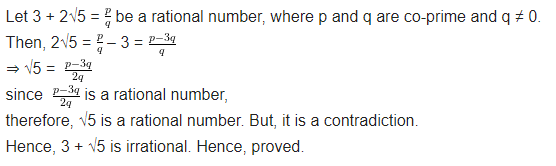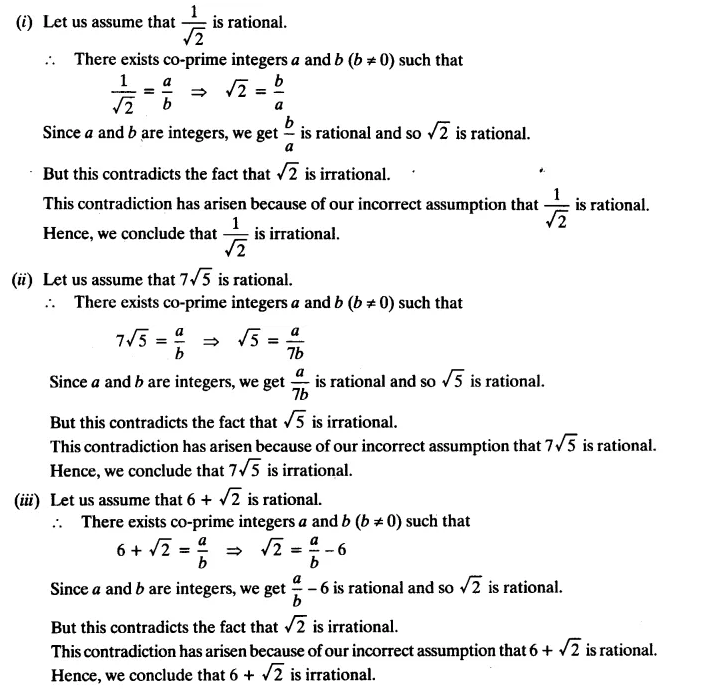# NCERT Solutions for Class 10 Maths Chapter 1 Real Numbers Ex 1.3

Get Free NCERT Solutions for Class 10 Maths Chapter 1 Ex 1.3 PDF.  Real Numbers Class 10 Maths NCERT Solutions are extremely helpful while doing homework.  Exercise 1.3 Class 10 Maths NCERT Solutions were prepared by Experienced ncert-books.in Teachers. Detailed answers of all the questions in Chapter 1 maths class 10 Real Numbers Exercise 1.3 provided in NCERT Text Book.

Topics and Sub Topics in Class 10 Maths Chapter 1 Real Numbers:

 Section Name Topic Name 1 Real Numbers 1.1 Introduction 1.2 Euclid’s Division Lemma 1.3 The Fundamental Theorem of Arithmetic 1.4 Revisiting Irrational Numbers 1.5 Revisiting Rational Numbers and Their Decimal Expansions 1.6 Summary

You can also download the free PDF of  Ex 1.3 Class 10 Real Numbers NCERT Solutions or save the solution images and take the print out to keep it handy for your exam preparation.

 Board CBSE Textbook NCERT Class Class 10 Subject Maths Chapter Chapter 1 Chapter Name Real Numbers Exercise Ex 1.3 Number of Questions Solved 3 Category NCERT Solutions

## NCERT Solutions for Class 10 Maths Chapter 1 Real Numbers Ex 1.3

NCERT Solutions for Class 10 Maths Chapter 1 Real Numbers Ex 1.3 are part of NCERT Solutions for Class 10 Maths. Here we have given Maths NCERT Solutions Class 10 Chapter 1 Real Numbers Exercise 1.3

Question 1.
Prove that √5 is irrational.
Solution:Question 2.
Show that 3 + √5 is irrational.
Solution:Question 3.
Prove that the following are irrational.Solution:error: Content is protected !!
+